For fitting thermal pollutions one wants to try the following model: $A_1 \exp(E t) + A_2 \exp(E \cdot (T-t)) \,.$ It has two amplitudes but only one energy. I have implemented this as TwoAmplitudesModel and restricted it to a single correlator. One could generalize this to fit a whole correlator matrix, but I cut the corners for now.

What we actually implement is the following to be consistent with the SingleModel: $\frac12 \left( A_1^2 \exp(E t) + A_2^2 \exp(E \cdot (T-t)) \right) \,.$

# Test with samplecf

The samplecf correlation function does not have thermal pollutions. Therefore we expect the model to recover the same amplitude for forward and backward part.

scf <- bootstrap.cf(samplecf)
plot(scf, log = 'y')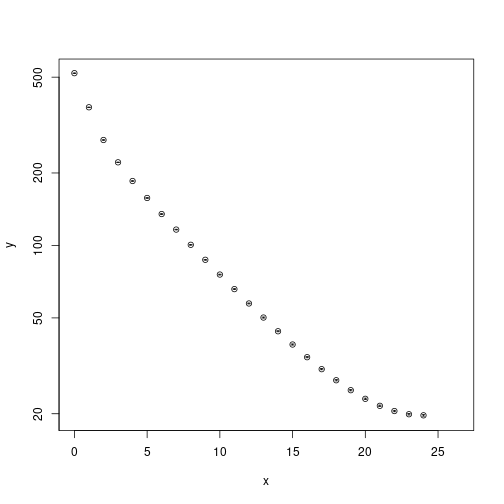fit_sample <- new_matrixfit(scf, 8, 22, model = 'single')
plot(fit_sample, log = 'y')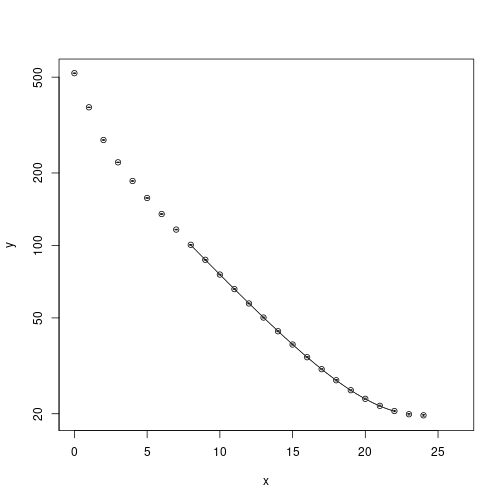residual_plot(fit_sample, ylim = c(1/1.05, 1.05))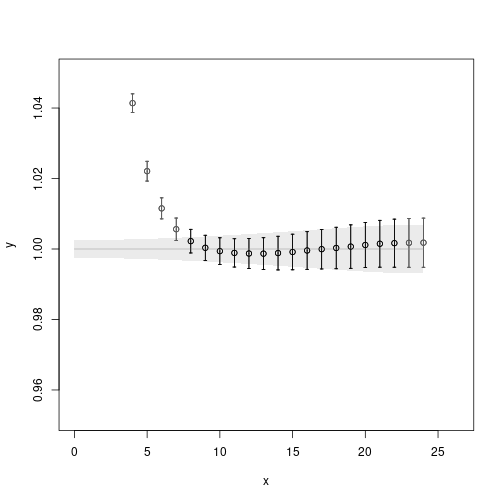fit_sample_2 <- new_matrixfit(scf, 8, 22, model = 'two_amplitudes')
plot(fit_sample_2, log = 'y')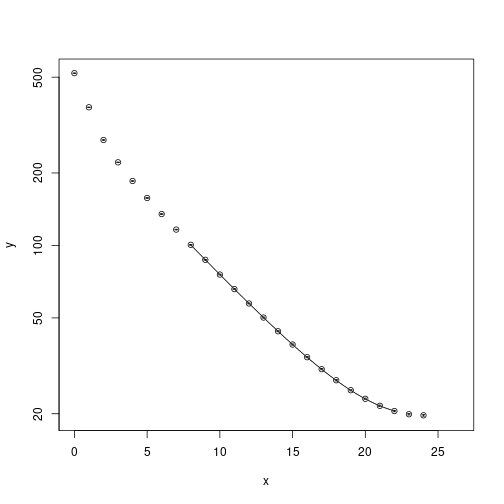residual_plot(fit_sample_2, ylim = c(1/1.05, 1.05))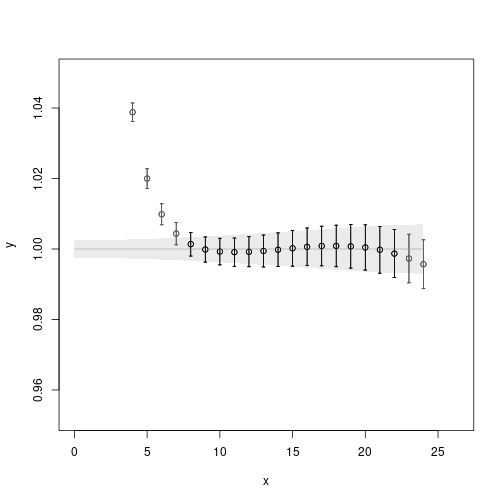Looking at the results from both fits, we see that the first fit produces $$(E, A)$$ which is reproduced by the second as $$(E, A_1, A_2)$$ pretty well:

mapply(tex.catwitherror, fit_sample$t0, fit_sample$se, with.dollar = FALSE)

## Loading required namespace: errors

##  "0.1446(3)" "25.15(3)"

mapply(tex.catwitherror, fit_sample_2$t0, fit_sample_2$se, with.dollar = FALSE)

##  "0.1450(3)" "25.20(3)"  "0.785(5)"


# Test with artificial data

We can make up an example which has different forward and backward amplitudes and constant noise.

extent_time <- 48
time <- seq(0, extent_time - 1, by = 1)
model_E <- 0.015
model_A1 <- 0.35
model_A2 <- 0.4
val <- 0.5 * model_A1^2 * exp(-model_E * time) + 0.5 * model_A2^2 * exp(-model_E * (extent_time - time))

plot(time, val,
main = 'Model data',
xlab = 't',
ylab = 'C(t)')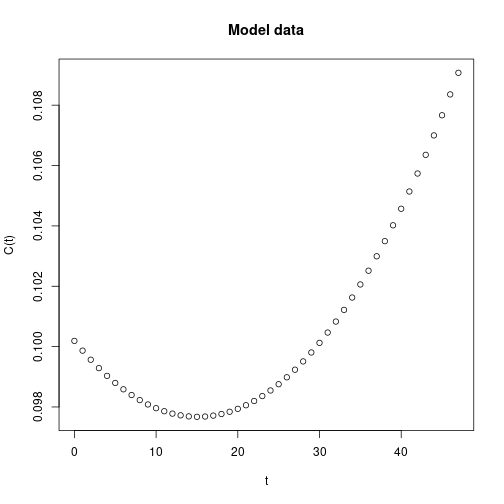measurements <- do.call(cbind, lapply(val, function (v) rnorm(400, v, 0.01)))

cf <- cf_orig(cf_meta(Time = extent_time), cf = measurements)
cf <- symmetrise.cf(cf)
cf_boot <- bootstrap.cf(cf)

plot(cf_boot, log = 'y')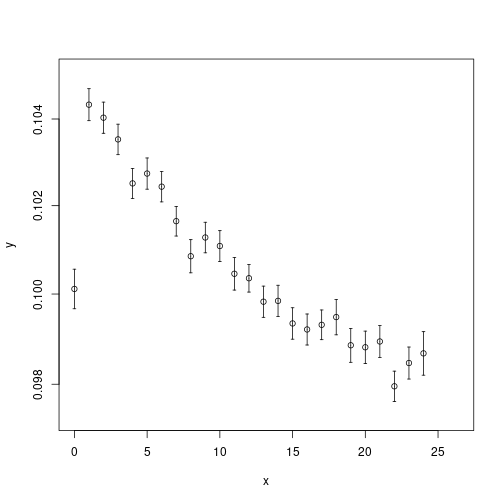We fit that using the new model and

fit <- new_matrixfit(cf_boot, 2, 23, model = 'two_amplitudes')
plot(fit, log = 'y')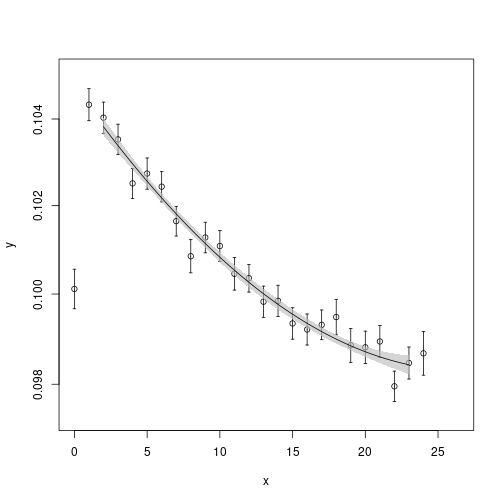residual_plot(fit)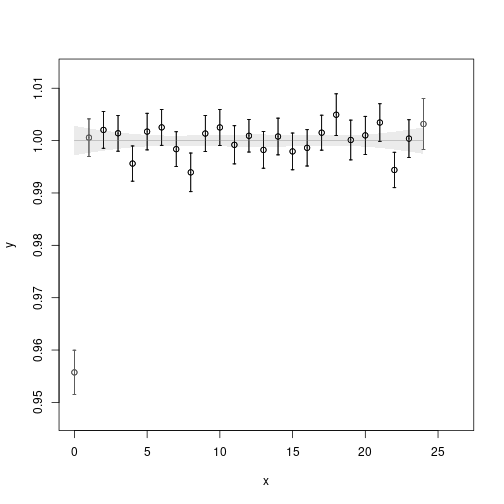Comparing with the input from the model gives a reasonable result:

print(c(model_E, model_A1, model_A2))

##  0.015 0.350 0.400

mapply(tex.catwitherror, fit$t0, fit$se, with.dollar = FALSE)

##  "-0.013(2)" "-0.262(2)" "-0.375(2)"